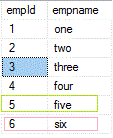top of page
• Jagan Mohan Reddy Rajidi

# SQL query to check two tables have identical data

Assume two tables emp1 and emp2 have same structure and same number of rows but one row is different in both tables as shown below.

```create table emp1(empId int,empname varchar(30))

insert into emp1 values(1,'one')
insert into emp1 values(2,'two')
insert into emp1 values(3,'three')
insert into emp1 values(4,'four')
insert into emp1 values(5,'five')

create table emp2(EmpId int,EmpName varchar(30))

insert into emp2 values(1,'one')
insert into emp2 values(2,'two')
insert into emp2 values(3,'three')
insert into emp2 values(4,'four')
insert into emp2 values(6,'six')```To cofirm both tables have identical data, Row count returned in below query should be same as number of rows in emp1 or emp2(row count of below query= row count of emp1= row count of emp2). Below query returns 6 rows(4 identical rows and 2 different rows) so two tables are not identical.

```select * From emp1
union
select * From emp2

```

Output:Row count in Emp1 is 5 but Row count in combined tables(emp1 union emp2) is 6. Hence both tables are not identical in data.

### Complete query to determine two tables are identical

Note: To check your two tables are having identical data, just replace tables emp1 and emp2 with your tables in below script and you will get the result.

```declare @rowcount_Table1 as int
select @rowcount_Table1=count(*) from emp1

declare @rowcount_Table2 as int
select @rowcount_Table2=count(*) from emp2

declare @rowcount_merge as int
select  @rowcount_merge=count(*) FROM
(select * from emp1
union
select * from emp2) T

if @rowcount_Table1>=@rowcount_merge  --Can also check as @rowcount_Table2=@rowcount_merge
begin
print 'Both tables have identical data'
Print 'Row Count in first table is ' + convert(varchar (3),@rowcount_Table1)
Print 'Row Count in second table is ' + convert(varchar (3),@rowcount_Table2)
Print 'Row count in merge of first and second tables ' +convert(varchar (3),@rowcount_merge)
end
else
begin
print 'Both tables are not having identical data'
Print 'Row Count in first table is ' + convert(varchar (3),@rowcount_Table1)
Print 'Row Count in second table is ' + convert(varchar (3),@rowcount_Table2)
Print 'Row count in merge of first and second tables ' +convert(varchar (3),@rowcount_merge)
end

```

Output: